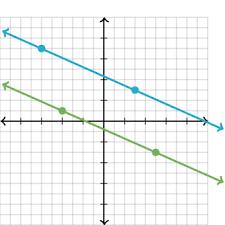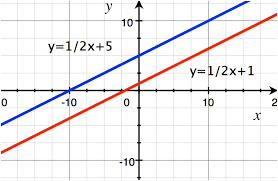Solution to the graph
Solution to the equation
Parallel, intersect or Same
Number of solutions
Solve by graphing
100no solution

100

( 1,5)      y= x + 4

y= 2x + 3

yes it is a solution

100

y= 2x + 4

y= 4+2x

Same Line

100no solutions

100

y=x+2

y=-x+4200(4,6)

200

(-2,4)   y= -x + 2

y= x + 2

No not a solution

200

y=-4x +8

y=-4x -8

Parallel Lines

200one solution

200

y=3x-5

y=-x-1300(2,2)

300

(-5, 6)   2x + 5y = 20

x - y =-11

yes it is a solution

300

y= -2x + 6

y= 3x +1

Intersecting Lines

300Infinite many

300

x-y=-1

3x+y=9400(1,-2)

400

(3,-7)    3x - 2y = 22

x -10=y

no it is not a solution

400

-3x = y + 3

y = 3x + 3

Same Line

400one solution

400

y=1/2x +5

y=1/2x + 1500(-2,6)

500

(-8,-4)    x -2y =0

2y=x

yes it is a solution

500
4x + 2y = 2

y + 2x = 4

Parallel Lines

500no solution

500

2x+2y=6

4x-6y+12Click to zoom